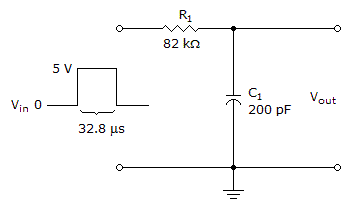# Electronics - Time Response of Reactive Circuits - Discussion

### Discussion :: Time Response of Reactive Circuits - General Questions (Q.No.1)

1.What voltage will the capacitor charge up to in the given circuit for the single input pulse shown?

 [A]. 3.15 V [B]. 4.3 V [C]. 4.75 V [D]. 4.9 V

Answer: Option B

Explanation:

No answer description available for this question.

 Phaneendra said: (Feb 14, 2011) f=1/RC Toff=1/f Ton+Toff=T;32.4+16.2=48.6 Ton/T=Von(drop) beacause we hav calucalate voltge during on state . V-Von=4.3v 5-0.66=4.3v

 Elkaa said: (Mar 26, 2011) Dear, If the timeconstant = RC is 16.4 usec, and the pulse is 32.4 usec, then I would expect that the capacitor charges up to Vin, as it has a lot more time then RC. Please confirm.

 Sreekumar said: (Jul 16, 2011) I agree with Elkaa, it will charge upto Vin.

 Nitesh said: (Sep 13, 2011) Vc(voltage across capacitor) = V0(1-e-t/to)

 Vasu said: (Oct 13, 2011) Can anyone explain this in brief please.

 Ganesh said: (Apr 21, 2012) I agree with elka.

 Mehta said: (Apr 25, 2012) Elkaa is totally wrong. It takes 5 timeconstant to charge upto Vin. So it won't charge upto Vin. Nitesh is right. In that equation substitute t = 32.8us and to = RC = 16.4us.

 Leena said: (Jun 22, 2013) Writing the kirchoff's current law you get Vin-iR-(1/C) inetgral (idt). On applying the laplace transform we get the equation, Vo(1-e-t/to). On substituting we get 4.3. Where to = 1/RC. Vo = 5.

 Arun said: (Oct 29, 2013) @Mehta is right. Given circuit is a low pass filter circuit. So output voltage is: Vo = Vin (1-e^(-t/RC)). T = 32.8 us. R = 82 k. C = 200 PF.

 Gaurav said: (Jan 16, 2016) T constant = rc = 82 k x 200 pf = 16.4 micro sec. Plus time = 32.8 micro sec. Trc = 32.8/16.4 = 2. 1 Trc = 63.3%. 2 Trc = 86%. So, output voltage = 5x(86/100) = 4.3 v.

 Padma said: (Aug 31, 2016) Yeah! @Gaurav is absolutely right.

 Prabhu said: (Nov 24, 2016) @Gaurav. Please explain about the 1 trc and 2 Trc calculation.

 Seema said: (May 7, 2017) Yeah! Please explain about 1trc and 2trc calculation.

 Kanha said: (Jul 7, 2017) (1-e^(-t/RC))=0.63 for t=RC and it is 0.86 for t = 2RC.

 Asha said: (Jul 20, 2017) Thank you @Gaurav.

#### Post your comments here:

Name *:

Email   : (optional)

» Your comments will be displayed only after manual approval.

#### Current Affairs 2021

Interview Questions and Answers